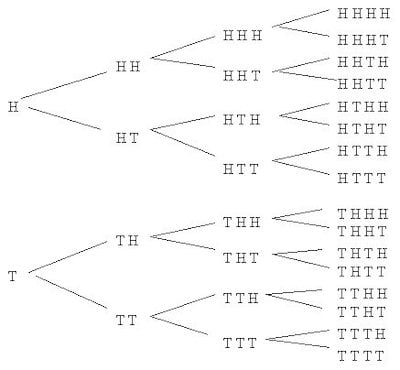Technical Article

# The Binomial Probability Distribution in Electrical Engineering

July 22, 2022 by Robert Keim

## This article, the first in a series, introduces the binomial distribution, a powerful statistical tool that is useful for reliability analysis, quality control, and other engineering applications.

Students and professionals alike might reach an impasse when they attempt to incorporate statistical methods into a real-life task. That’s why I like the binomial distribution as it provides a prepackaged framework for practical, effective statistical analysis. A high-level example can be seen below in Figure 1.##### Figure 1. The binomial distribution can be used to investigate the likelihood of a lightning strike. Image used courtesy of Dave Young [CC BY 2.0]

Your primary tasks when deploying the binomial distribution are to confirm that you are in fact working in a binomial setting and then to integrate your particular setting into the binomial framework. After that, you can determine probabilities and perform statistical analysis using straightforward formulae and simple calculator tools.

### The Binomial Setting

You can apply the binomial distribution if your situation fulfills the following three conditions:

1. The number of trials is fixed. The term “trial” here is much less restrictive than it sounds; more on this below.
2. Each trial has only two possible outcomes, called success and failure.
3. The trials are identical and independent, such that the probability of success is the same for every trial.

### Binomial Distribution Trials—What is a Trial?

The term “trial” suggests a deliberate action with an uncertain outcome, such as flipping a coin or administering a pharmaceutical product. This narrow interpretation confines the binomial distribution to a small and, for engineers, potentially irrelevant subset of analytical scenarios. The key to success (no pun intended) with the binomial distribution is to interpret “trial” as any event, object, person, group, or place to which a true/false condition can be applied.

Here are a couple of examples:

• Let’s say you’re investigating study habits among engineering students. You know from previous research that 20% of engineering students nationwide do not study on the weekends, and you want to analyze a random sample of 45 engineering students from a specific university. Each student in the random sample is a “trial,” and the number of “trials” is 45 (i.e., the same as the number of students in the sample).
• If you’re taking a multiple-choice exam and guessing, each question on the exam is a trial.
• There are 12 software companies near your house. If you’re calculating the probability, based on national statistics, of finding at least one nearby software company that offers a four-day work week, the software companies are the trials, and the number of trials is 12.

Keep in mind that we’ll work through some examples in subsequent articles, which will allow you to continue strengthening your understanding of trials in the binomial setting.

#### Bernoulli Trial

Before we move on, I want to briefly mention that trials of this type—only two possible outcomes, with the same probability of success every time—are called Bernoulli trials. This term was named after Jacob Bernoulli, a seventeenth-century mathematician. The Bernoulli trial is associated closely, but not exclusively, with the binomial distribution. The trials pertaining to a different distribution called the geometric distribution are also Bernoulli trials.

### Establishing the Probability of Success or Failure

In everyday conversation, the words “success” and “failure” have strong positive and negative connotations. It’s important to understand that those connotations are nonexistent when we’re discussing and applying the binomial distribution.

In a binomial setting, success and failure are neutral identifiers that you can freely attach to something good, bad, or indifferent. The conventional approach is to define the “outcome of interest” as a success; this can lead to awkward situations in which “success” refers to the death of a subject, a circuit-design error, or a system failure.

After establishing the meaning of success and failure in your particular binomial scenario, you need to determine the probability of success (denoted by p) or the probability of failure (denoted by q).

Below are some key points to keep in mind:

• You don’t use the binomial distribution to find the probability of success or the probability of failure. Rather, these probabilities are prerequisite information obtained from published research, prior observation, probability theory, or reasonable conjecture. The binomial distribution helps us to apply existing knowledge of success and failure to a specific analytical scenario. One example scenario is flipping a coin (Figure 2).##### Figure 2. A coin toss is an example of a binomial experiment in which the probability of success, p = 0.5, is known from theory (and from experience). Image used courtesy of Norman Fenton

• Comprehensive application of the binomial distribution requires both p and q. Above I indicated that you need only p or q, and that’s because, in a binomial setting, the probability of success and the probability of failure always sum to one. If you know p, you also know q; if you know q, you also know p.

$$p+q=1\ \ \ \ \ \Rightarrow\ \ \ \ \ p=1-q,\ \ \ \ \ q=1-p$$

• Sometimes you can shoehorn an ostensibly refractory situation into the binomial setting by interpreting multiple outcomes as one collective outcome. For example, earlier I mentioned guessing on a multiple-choice exam. Multiple-choice questions usually have at least four options. How can we reconcile four possible outcomes with the two-outcome paradigm of a Bernoulli trial? All we need to do is define a correct outcome as a success and any incorrect outcome as a failure.

In this article, we discussed the general concept of binomial distribution as a real-life analytical tool, and we covered the characteristics of the binomial setting. In the next article, we’ll explore the process of calculating probabilities and summary statistics with the binomial distribution, and we’ll work through an example.